Question

A vertical solid steel post of diameter d = 20 cmand length L = 2.80 m is required to support a load of mass m = 8500 kg . You can ignore the weight of the post. Take free fall acceleration to be g=9.8m/s2.

A) What is the stree in the post?

B) What is the strain in the post?

C) What is the change in the post's length when the load is applied?

(Express all answers using 2 sigfigs)

given data

A vertical solid steel post of diameter d = 20 cm

and length L = 2.80 m

load of mass m = 8500 kg .

A vertical solid steel post 20 cm in diameter and 2.80m long is required to support a load of 8500 kg. You may ignore the weight of the post.

The stress is the ratio of the external force to the cross sectional area.
The strain is the ratio of the change of length to the original length.
Young’s modulus = stress/ strain

A)What is the stress in the post when the load is applied?
The weight of the 7900 kg load is the force = 8500 * 9.8
Area = π * r^2 = π * 0.10^2
Stress = (8500 * 9.8) ÷ (π * 0.10^2) = 2.65* 10^6 Pascals
So, stress is pressure!

B)What is the strain in the post when the load is applied?
Strain = stress ÷ Young’s modulus = 2.65* 10^6 ÷ 200 * 10^9 = 1.325*10^-5

C)What is the change in the post's length when the load is applied?
The strain is the ratio of the change of length to the original length.
Increase of length = Original length * strain = 2.8 * 1.325*10^-5 = 3.71* 10^-5 m

#### Earn Coins

Coins can be redeemed for fabulous gifts.

Similar Homework Help Questions
• ### A vertical solid steel post of diameter d = 22 cm and length L = 2.30...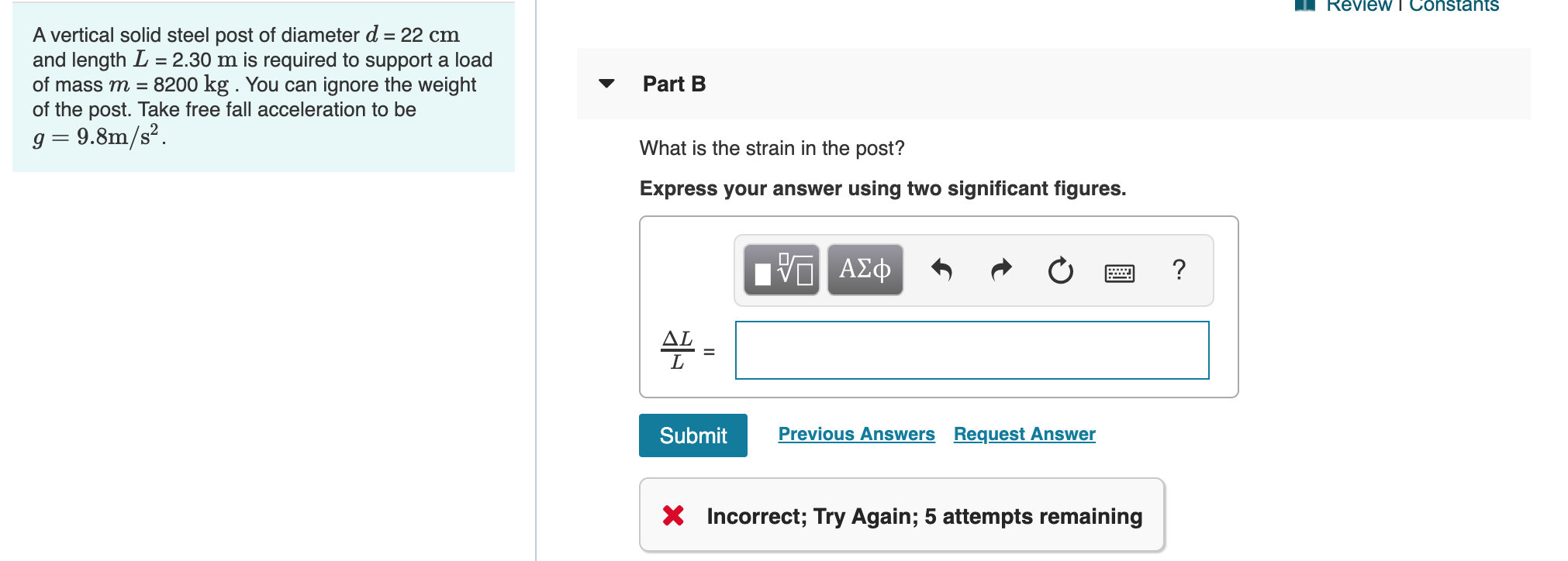A vertical solid steel post of diameter d = 22 cm and length L = 2.30 m is required to support a load of mass m = 8200 kg . You can ignore the weight of the post. Take free fall acceleration to be g=9.8m/s2. B. What is the strain in the post? Express your answer using two significant figures. C. What is the change in the post's length when the load is applied? Express your answer using two significant...

• ### A vertical solid steel post of diameter d = 27 cm and length L = 3.00 m is required to support a load of mass m = 8000 kg . You can ignore the weight of the post. Take free fall acceleration to be g=9...

A vertical solid steel post of diameter d = 27 cm and length L = 3.00 m is required to support a load of mass m = 8000 kg . You can ignore the weight of the post. Take free fall acceleration to be g=9.8m/ s 2 Part A What is the stress in the post? Express your answer using two significant figures. Part B What is the strain in the post? Express your answer using two significant figures. Part...

• ### a vertical solid <Ch 11 HW Exercise 11.30 A vertical solid steel post of diameter d...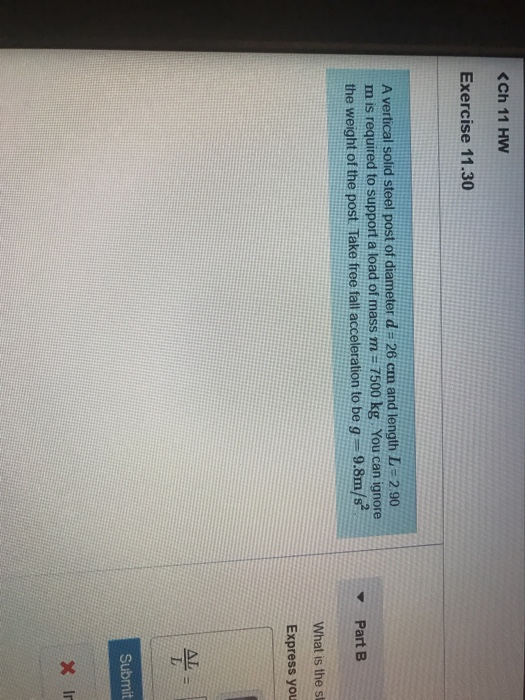a vertical solid <Ch 11 HW Exercise 11.30 A vertical solid steel post of diameter d = 26 cm and length L=2.90 m is required to support a load of mass m = 7500 kg You can ignore the weight of the post Take free fall acceleration to be g = 9.8m/s Part B What is the st Express you Submit X ir 2.90 gnore Part A What is the stress in the post? Express your answer using two significant...

• ### Segment AB is a solid steel shaft that is 2 m in length, 0.1 m in...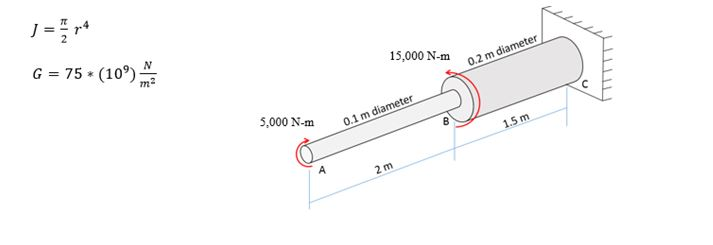Segment AB is a solid steel shaft that is 2 m in length, 0.1 m in diameter, and has an applied torque of 5,000 N∙m in the clockwise direction. Segment BC is a solid steel shaft that is 1.5 meters in length, 0.2 meters in diameter, and has an applied torque of 15,000 N∙m in the counter clockwise direction. a.)    Determine the maximum shear stress, in MPa, in the shaft b.) Determine the angle of twist, in degrees, in section...

• ### 17.3 A solid circular steel column having a d-150 mm diameter is subjected to a centric...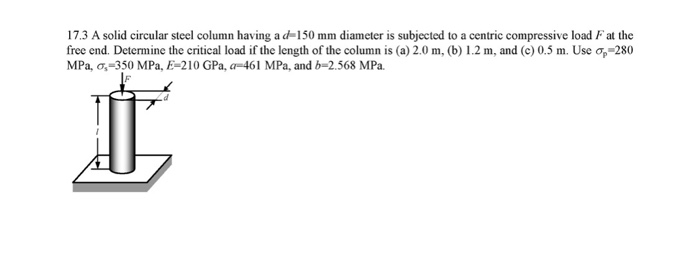17.3 A solid circular steel column having a d-150 mm diameter is subjected to a centric compressive load Fat the free end. Determine the critical load if the length of the column is (a) 2.0 m, (b) 1.2 m, and (c) 0.5 m. Use op-280 MPa, 0,=350 MPa, E-210 GPa, a-461 MPa, and b=2,568 MPa.

• ### A solid steel bar of circular cross section has diameter d = 40 mm, length L...

A solid steel bar of circular cross section has diameter d = 40 mm, length L = 1.3 m and shear modulus of elasticity G = 80 GPa. The bar is subjected to torques T acting at the ends. If the torques have magnitude T = 340 Nm, what is the maximum shear stress in the bar? What is the angle of twist between the ends? If the allowable shear stress is 42 MPa and the allowable angle of twist...

• ### A steel wire, of length L and diameter D, has a resistance of 100 Ω. What...

A steel wire, of length L and diameter D, has a resistance of 100 Ω. What current would flow through a silver wire of the same diameter and the same length if a 20.0 V potential difference were applied across its length?

• ### spring-2010 5. (a) A steel wire of diameter 0.60 mm and length 1.5 m. support A...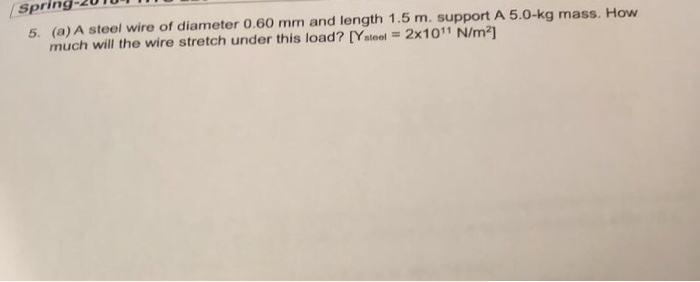spring-2010 5. (a) A steel wire of diameter 0.60 mm and length 1.5 m. support A 5.0-kg mass. How much will the wire stretch under this load??Ystee-2x 1011 N/m2]

• ### 75 mm 1.5 m T = 48 N·m 2 m 150 mm Two solid steel 20-mm-diameter...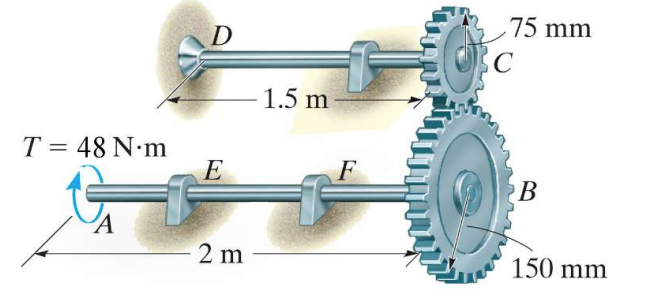75 mm 1.5 m T = 48 N·m 2 m 150 mm Two solid steel 20-mm-diameter shafts are coupled together using the perfectly meshed gears shown. Shaft DC is fixed to the wall at D and supported with a frictionless journal bearing. Shaft EF is supported with two frictionless journal bearings. The modulus of rigidity of steel is 80 GPa. Before the torque is applied, the angle of twist in each shaft is zero, and the angle of rotation of...

• ### A steel pipe column has a length L=4.25 m and has tubular cross section with inside...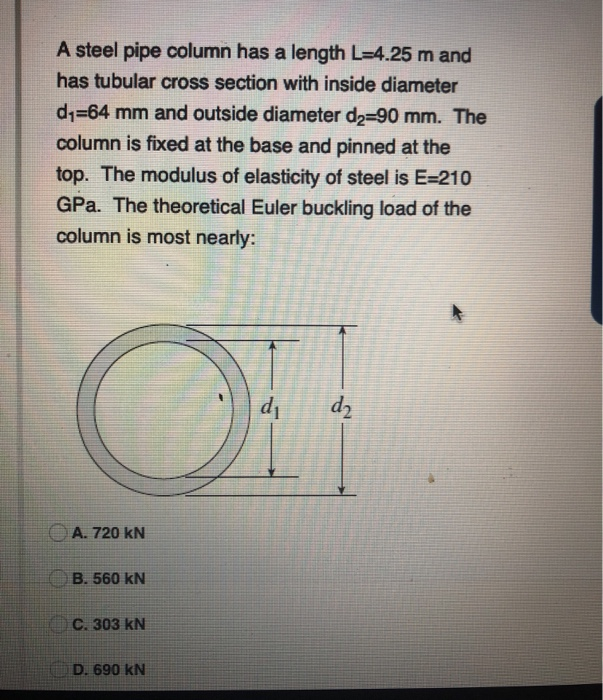A steel pipe column has a length L=4.25 m and has tubular cross section with inside diameter dy=64 mm and outside diameter d2=90 mm. The column is fixed at the base and pinned at the top. The modulus of elasticity of steel is E=210 GPa. The theoretical Euler buckling load of the column is most nearly: dy dz A. 720 KN B. 560 kN C. 303 kN D. 690 KN The steel post has a length L=4 m and carries...